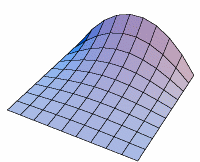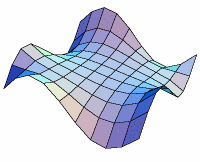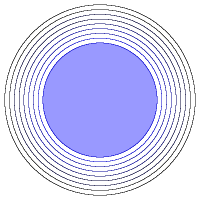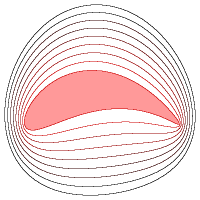Fall 2003

# Mathematics Math21b Fall 2003

## Linear Algebra and Differential Equations

Office: SciCtr 434
Email: knill@math.harvard.edu# Laplace equation

(a partial differential equation)

## Laplace equation and Dirichlet probem

A function f(x,y) which satisfies the Laplace equation fxx + fyy=0 is called harmonic. In other words, they are in the kernel of the linear operator T=Dxx + DyyThe Dirichlet problem for a region G in the plane is to find a function f(x,y) which is harmonic inside the region and which takes prescribed values at the boundary. The Dirichlet problem can easily be solved with Fourier theory on squares: one first solves the problem, where the function is nonzero only on one side of the square, then writes the general solution as a superposition of four such solutions.## Conformal transformationOne can find solutions to the Dirichlet problem for a wider class of regions by conformal transformations. These are transformations which preserve the angles and have the property that the transplanted function is harmonic if the original function is harmonic. One can obtain conformal transformations using complex function (x,y) -> (u,v): if z=x+iy and F(z) is a polynomial in z and F(z)=u+i v, then the map is conformal. For example, to solve the Dirichlet problem outside a wing, one can transform the circle into the wing using a conformal transformation. Such methods are relevant in engeneering.## Quantum evolutionThe Eigenvalue problem for a region G in the plane asks to find explicit formulas for the eigenvalues and eigenvectors of the linear operator T=Dxx + Dyy on smooth functions in G which vanish on the boundary of G. For regions in which the billiard ball problem is explicitly solvable, one expects the eigenvalues and eigenfunctions of the linear operator T=Dxx + Dyy to be computable explicitly also. The PDE ft(t,x,y) = i T f(t,xy,z) is called the Schrödinger equation for a free particle in the region G Knowing the complex valued function f(t,x,y) allows to compute the probability that t>e particle is inside some region D by integrating |f|2 over D and dividing this by the integral of |f|2 over G. Because for eigenfunctions T f = L f one can easily find the solution f(t,x,y) = exp(i L t) f(0,x,y), the evolution of a general function f is obtained by writing f as a linear combination of eigenfunctions and summing up the evolved eigenfunctions.

Back to the main page